Approximating a distribution with an exponential family using a custom loss function

Exponential Family is a family of (normalized) functions which is log-linear. In other words, if we take logarithm of each point, we will get a vector space. Various statistical distribution families like Normal, Gamma, Beta are examples of exponential families.
Here I look exponential families of the form 1/(1+Exp[polynomial of degree n]), and give a procedure to fit such exponential family to given distribution using a custom loss function.

The function

In:=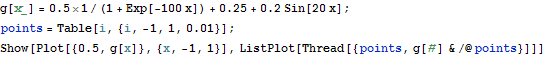Out=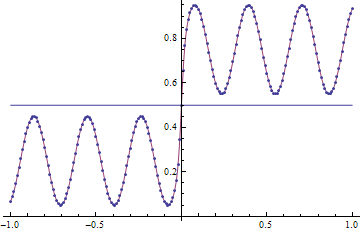Losses

Log - loss truncated to avoid division by 0 errors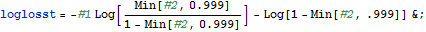In:=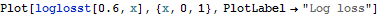Out=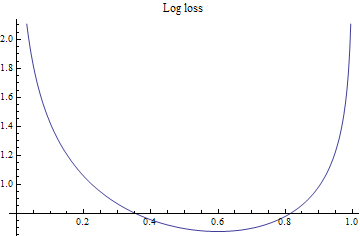In:=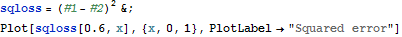Out=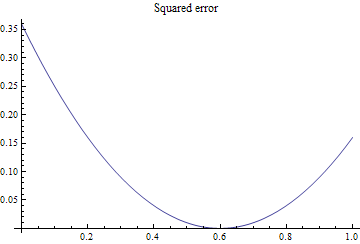Fitter

In:=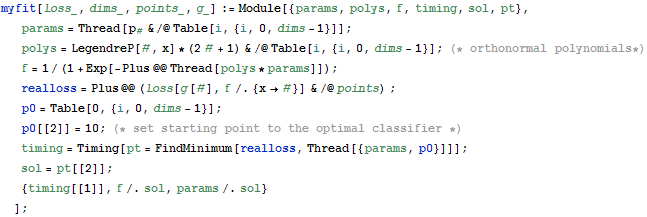Demo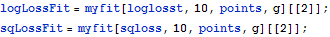In:=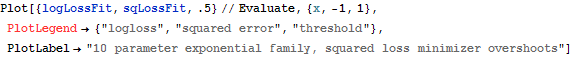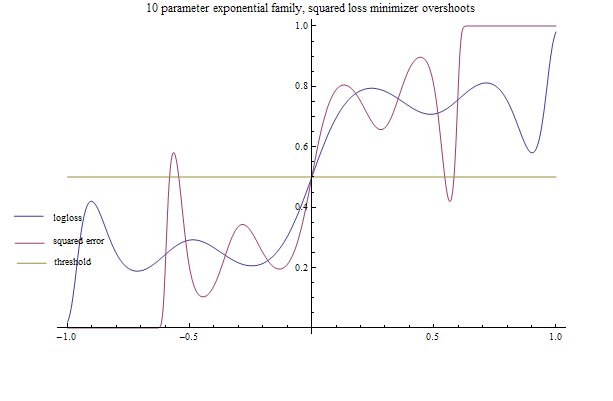Rank-1 decomposition to improve GraphPlot

Consider applying Thorp shuffle to 4 cards.This can be viewed as a random walk on a graph with 4! nodes which we can visualize using GraphPlot. The graph is highly regular, however, GraphPlot doesn' t detect this regularity, and the result looks quite jumbled. We can fix this by using rank-1 decomposition (SVD) to suggest a natural grouping of nodes. Group nodes together if they are source nodes in the same rank-1 component of the adjacency matrix, and a nice octahedral structure emerges.

In:=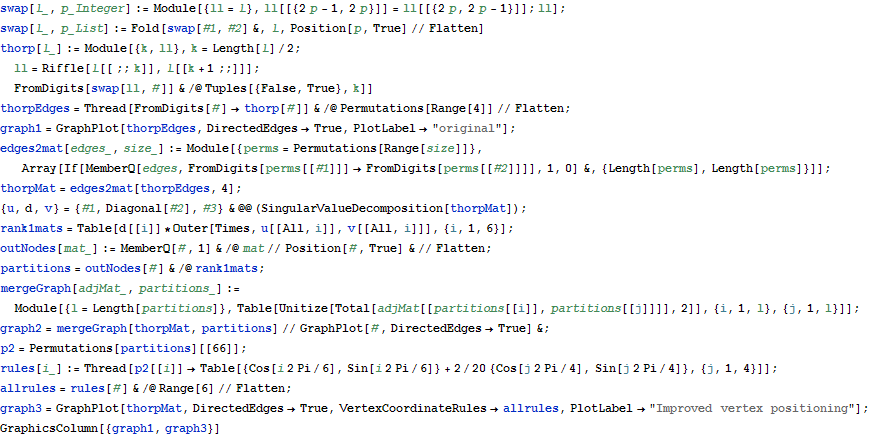Out=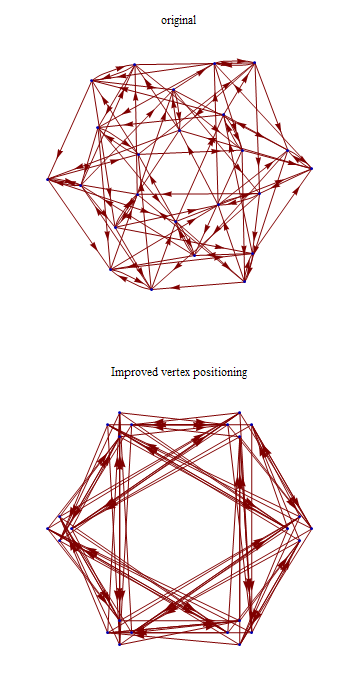Closed form for Logistic Regression

The code below constructs and solves maximum likelihood equations for saturated Logistic regression model over k binary variables.

In:=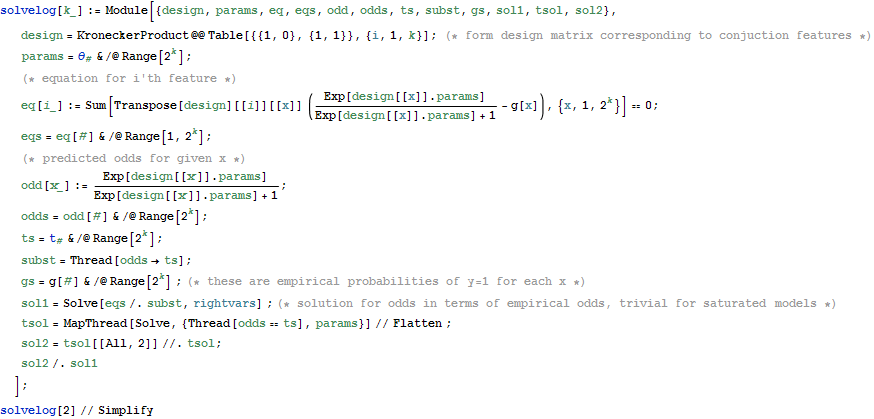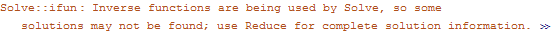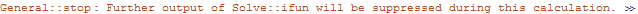Out=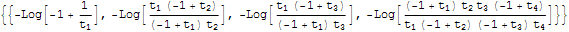Visualizing Fisher' s Linear Discriminant

Fisher' s linear discriminant attempts to find an optimal separating hyperplane by maximizing the ratio of between - class scatter to within - class - scatter. The dynamic section shows separating boundary + class outlines

(Debug) In:=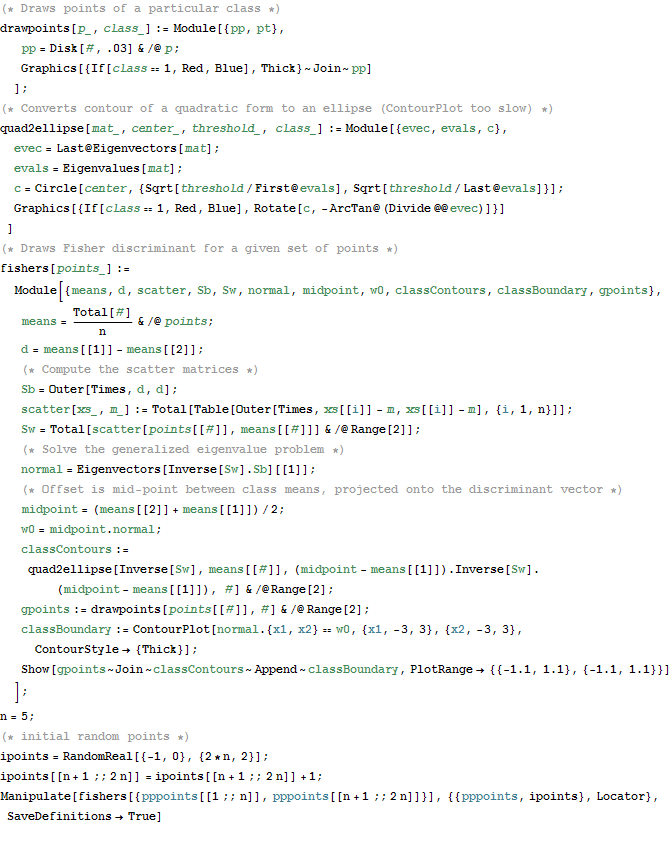(Debug) Out=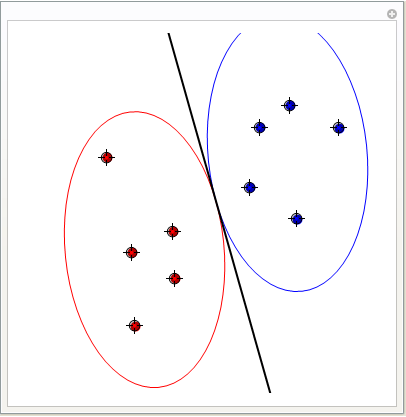Computing Effective Resistance between 2 points in a network

I programmed this in order to access a Russian physics forum which requires you to find resistance between end - nodes of a Wheatstone bridge in order to gain access. In the process of researching this issue I found 7 other ways to compute this resistance which I' d like to implement sometime

Out=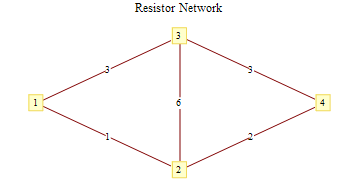In:=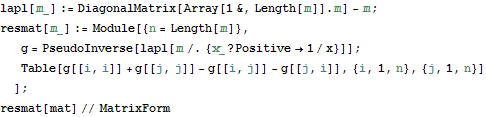Out//MatrixForm=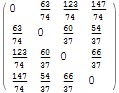MaxEnt distribution with a skewed sufficient statistic

If we maximize entropy subject to moment constraints we can get Normal, Exponential, Gamma and a few other distributions, depending on a particular form of constraints. If our constraints are on first, second and third moments, then there' s no closed form for the maxent distribution. Below I find the maxent solution numerically. I used NullSpace to turn this into lower-dimensional problem, LinearSolve to find a starting solution satisfying positivity constraints, and FindMaximum for the final solution

In:=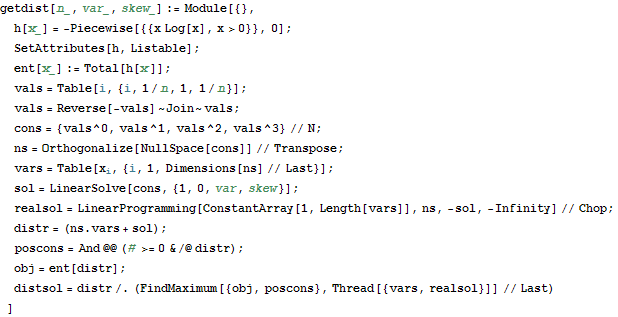In:=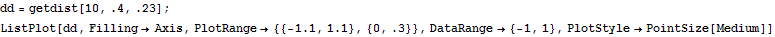Out=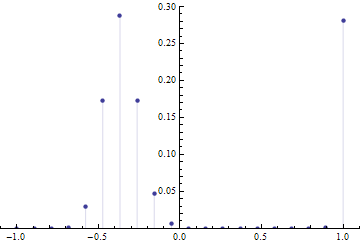Density Plot of the magnitudes in Farey Sequence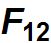Actually of the logarithm of the magnitudes. Self-similar structure emerges

In:=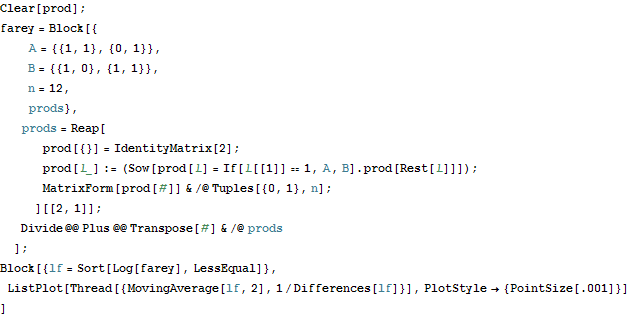Out=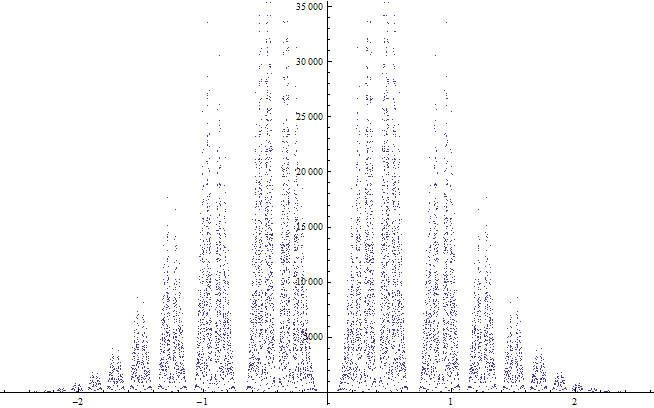Generating a random doubly stochastic matrix

Method 1, iterative proportional fitting

This method normalizes rows/columns in alternation. It converges with probability one and produces a doubly stochastic matrix that is closest to the random starting matrix in KL - divergence

In:=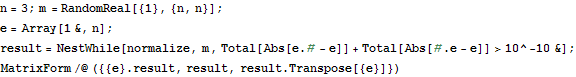Out=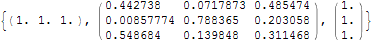Method 2, MCMC (From paper by Diaconis, "Algebraic Algorithms For Sampling ...")

This method constructs a Markov chain which randomly perturbs the matrix at each step, without destroying double - stochasticity. It is initialized with a random permutation matrix.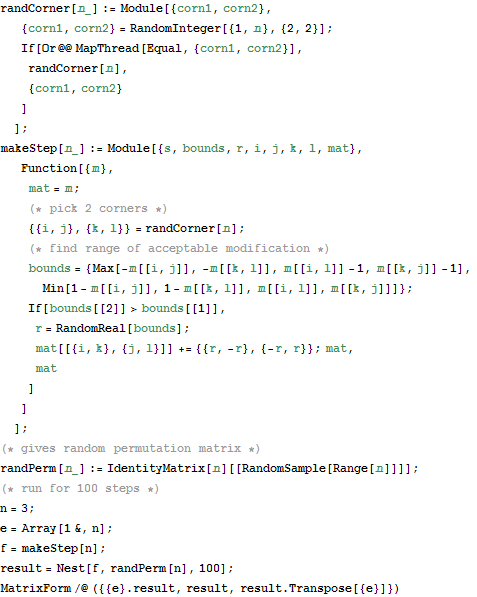Out=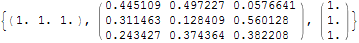Visualize Gibbs sampling of a Hard - Core Lattice Gas model

Hard - core model pretends each molecule can occupy only one of a set of fixed sites on a lattice, and neighbouring sites can not be simultaneously occupied. Code below implements Gibbs sampling to draw random configurations from this model. Visualization shows current configuration, and plot of number of sites occupied in previous iterations of MCMC run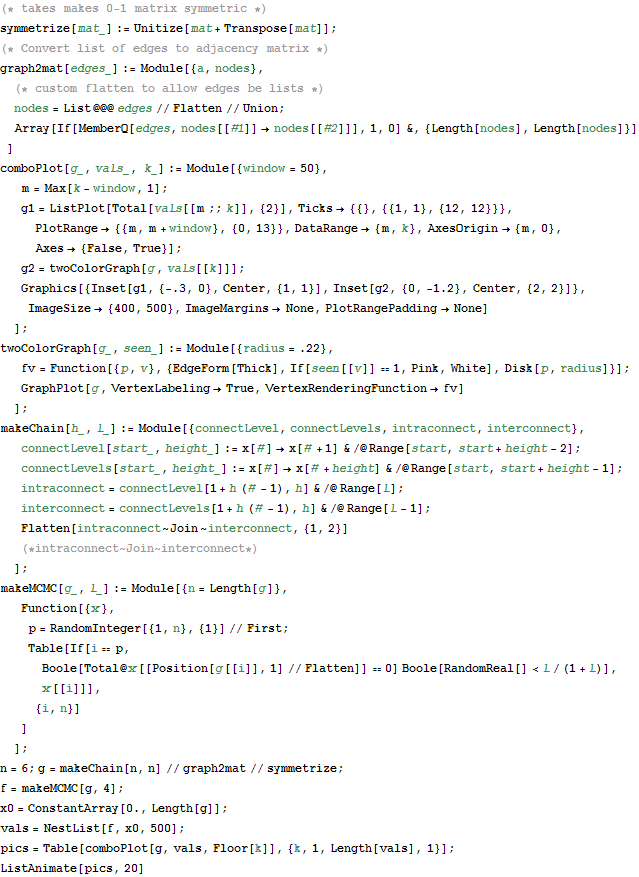Out=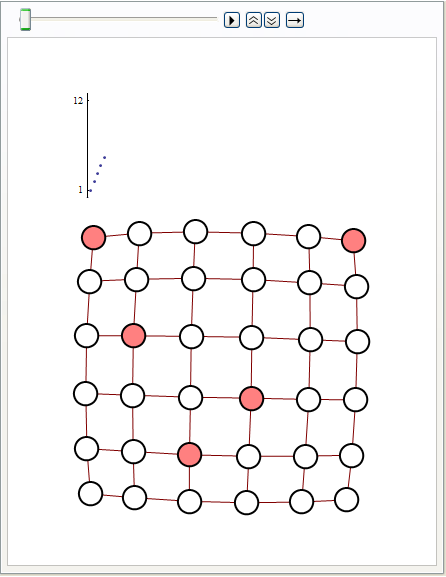Bethe approximation for Ising spin-glass

The code below computes a sequence of Bethe - Peierls - like approximations for computing mean magnetization for each site in a planar Ising spin - glass model. The idea for the approximation scheme came from Alan Sokal' s "The Repulsive Lattice Gas ... " paper and is based on limited memory self-avoiding walks. For the simple graph below, memory-5 walks recover magnetization values exactly. The graph plots estimated magnetization values for 5 nodes, last number compares approximate result with exact, obtained by explicitly summering over all configurations

In:=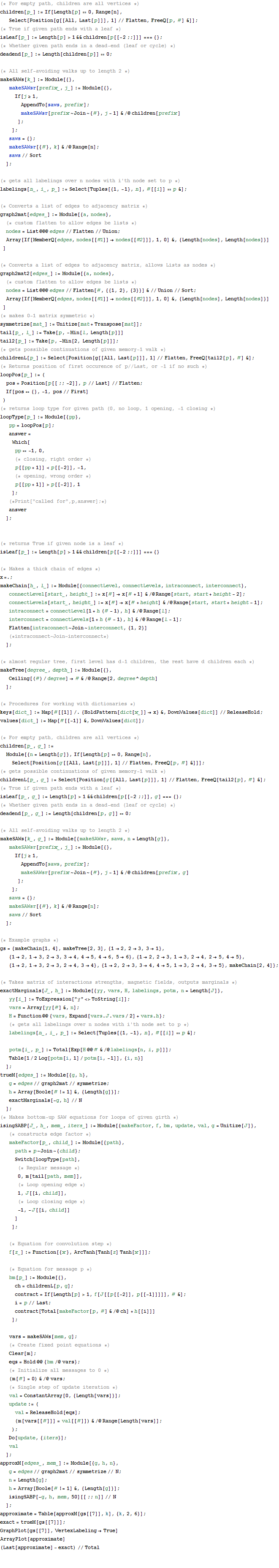Out=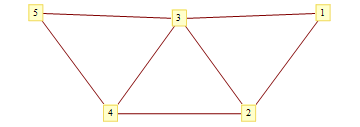Out=Out=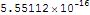In:=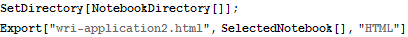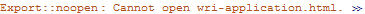Out=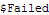Created by Wolfram Mathematica 6.0  (10 March 2008)